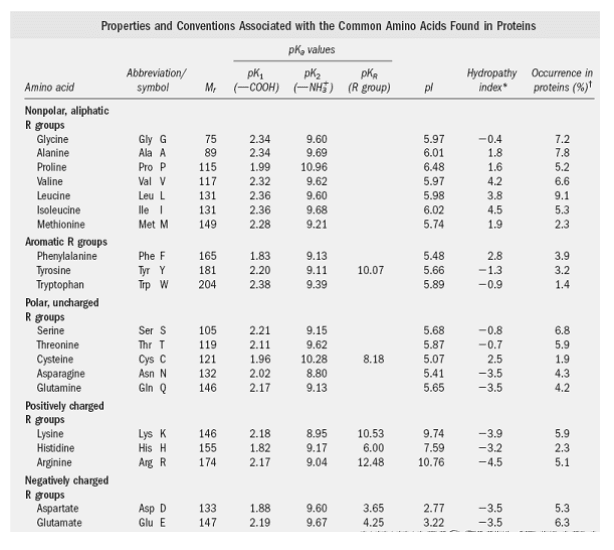# What is Zwitter Ion and Isoelectric point

Amino acids have at lease two ionizable groups, i.e., -COOH and -NH2+, of which the former dissociates more easily than the latter. at physiological pH (7.3), the carboxyl group exists in ionized form whereas the amino group remains undissociated and thus retains a positive charge. This doubly charged molecule of amino acid containing a negative and a positively charged group is electrically neutral and is called Zwitter ion.

Index of the Article

## What is Zwitter Ion?

In Strong acid conditions (around pH 1.1) the alpha-COOH group remains undissociated. However when the pH is raised and reaches around pH 3.0, the proton from the carboxyl group is leaving a -COO- group. This is called the pK of the acid group and at this pH, dissociated and undissociated species are found in equal concentrations.

Similarly, when the pH is further raised to around 9.8 the amino group is dissociated – this pH is called the pK of an amino group. Thus, dicarboxylic acid like glutamic acid can have three pK values (two for carboxyl groups and one for the amino group) and four types of charged molecules as shown below.The name zwitter is derived from the German word which means “hybrid”. Zwitterion (or) dipolar ion is a hybrid molecule containing positive & negatively ionic groups. Basically the proton shifts from carboxyl group to amino group of the self-molecule at normal pH cellular levels.

The amino acids rarely exist in a neutral form with free carboxyl (-COOH) and free amino (-NH2) groups. In strongly acidic pH (low pH), the amino acid is positively charged (Cation). Each amino acid has a characteristic pH at which it carries both positive and negative charges and exists as a Zwitter ion.

## What is an Isoelectric Point (pI)?

At which pH, amino acids don’t migrate towards cathode (or) anode under the influence of electric field, is simply called “Isoelectric point”.

Isoelectric pH is defined as the pH which a molecule exists as a Zwitter ion (or) dipolar ion and carries no net charge. Thus, the molecule is electrically neutral, but the charge will cancel each other.

### Isoelectric Points of Some Important Proteins:

• Isoelectric point of lysozyme (Egg white) – 11.0
• Isoelectric point of Insulin (Bovine) – 5.4
• Isoelectric point of Myoglobin (Horse) – 7.0
• Isoelectric point of Serum Albumin (Human) – 4.9
• Isoelectric point of Ribonuclease (Bovine) 7.8 / 9.5

## Solubility and buffering capacity will be minimum at isoelectric pH

• To such a solution if we add hydrochloric acid drop by drop, at a particular pH, 50% of the molecules are in cationic form and 50% in zwitterionic form. Thus, pH is pK1 (with regard to –COOH). If more HCl is added, more molecules become cationic in nature and solubility increases.
• If we titrate the solution from pI with NaOH, molecules acquire the anionic form. When 50% of molecules are anionic that pH is called pK2.
• The isoelectric point (pI) for more amino, monocarboxylic amino acids can be calculated:

pK1 + pK2

pI =—————-

2

• The isoelectric point (pI) for more Acidic amino acids can be calculated:

pK1 + pK3

pI =—————-

2

• The isoelectric point (pI) for more amino, monocarboxylic amino acids can be calculated:

pK2 + pK3

pI =—————-

2

pK1=Dissociation constant of (-COOH) group

pK2=Association constant of (-NH2) group

pK3=Dissociation (or) Association constant of “R” side chain

## Problems and Solutions on Zwitter ion and Isoelectric Point

1. Calculate the Isoelectric point of Glycine? (pK1=2.4; pK2=9.8)

Ans: Glycine is a neutral and optically inactive amino acid. According to isoelectric point concept, the given formula will be applied;

pK1 + pK2                                   2.4 + 9.8

pI =—————-       =      —————— = 6.1

2                                        2

The isoelectric point of the Glycine is 6.1

2. Calculate the Isoelectric point of Lysine? (pK1 = 2.2, pK2=8.9; pK3=10.5)

Ans: Lysine is basic amino acids (diamino monocarboxylic acid). According to isoelectric point concept, the given formula will be applied:

pK1 + pK3                   2.2 + 10.5

pI =—————- = ————– = 9.7

2                               2

The isoelectric point of the Lysine is 9.7

Lysine has Guanidinium group in the ‘R’ side chain of Lysine.### Related Posts

This site uses Akismet to reduce spam. Learn how your comment data is processed.